# Recitation on analysis of algorithms Formal definition of

• Slides: 20Recitation on analysis of algorithms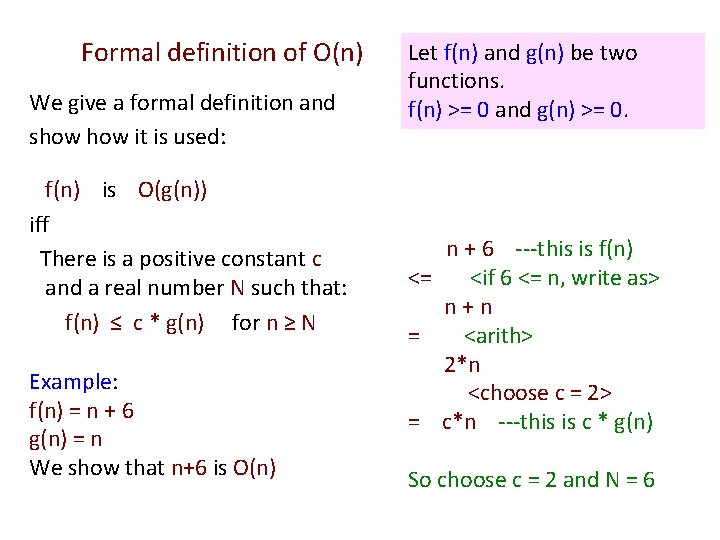Formal definition of O(n) We give a formal definition and show it is used: f(n) is O(g(n)) iff There is a positive constant c and a real number N such that: f(n) ≤ c * g(n) for n ≥ N Example: f(n) = n + 6 g(n) = n We show that n+6 is O(n) Let f(n) and g(n) be two functions. f(n) >= 0 and g(n) >= 0. n + 6 ---this is f(n) <= <if 6 <= n, write as> n+n = <arith> 2*n <choose c = 2> = c*n ---this is c * g(n) So choose c = 2 and N = 6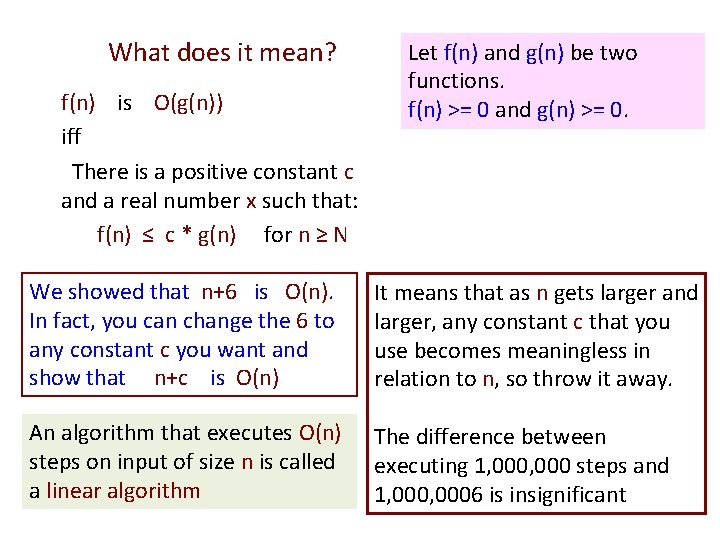What does it mean? f(n) is O(g(n)) iff There is a positive constant c and a real number x such that: f(n) ≤ c * g(n) for n ≥ N Let f(n) and g(n) be two functions. f(n) >= 0 and g(n) >= 0. We showed that n+6 is O(n). In fact, you can change the 6 to any constant c you want and show that n+c is O(n) It means that as n gets larger and larger, any constant c that you use becomes meaningless in relation to n, so throw it away. An algorithm that executes O(n) steps on input of size n is called a linear algorithm The difference between executing 1, 000 steps and 1, 0006 is insignificant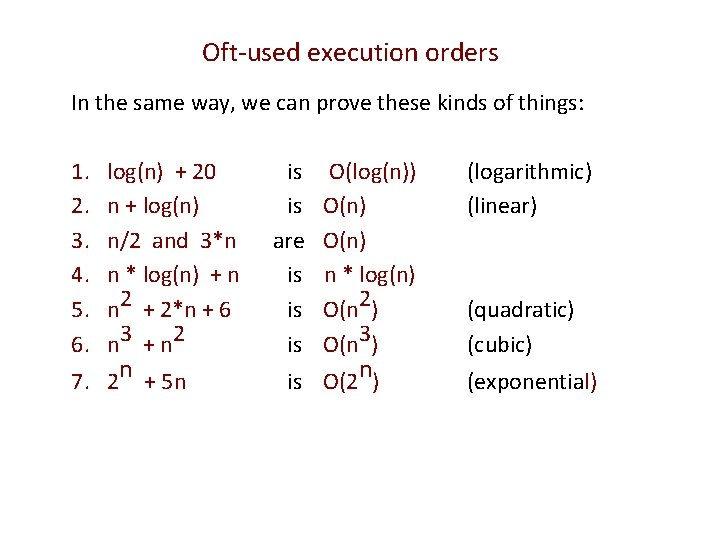Oft-used execution orders In the same way, we can prove these kinds of things: 1. 2. 3. 4. 5. 6. log(n) + 20 n + log(n) n/2 and 3*n n * log(n) + n n 2 + 2*n + 6 n 3 + n 2 7. 2 n + 5 n is is are is is is O(log(n)) O(n) n * log(n) O(n 2) O(n 3) is O(2 n) (logarithmic) (linear) (quadratic) (cubic) (exponential)Understand? Then use informally 1. 2. 3. 4. 5. 6. log(n) + 20 n + log(n) n/2 and 3*n n * log(n) + n n 2 + 2*n + 6 n 3 + n 2 7. 2 n + 5 n is is are is is is O(log(n)) O(n) n * log(n) O(n 2) O(n 3) is O(2 n) (logarithmic) (linear) (quadratic) (cubic) (exponential) Once you fully understand the concept, you can use it informally. Example: An algorithm executes (7*n + 6) / 3 + log(n) It’s obviously linear, i. e. O(n) steps.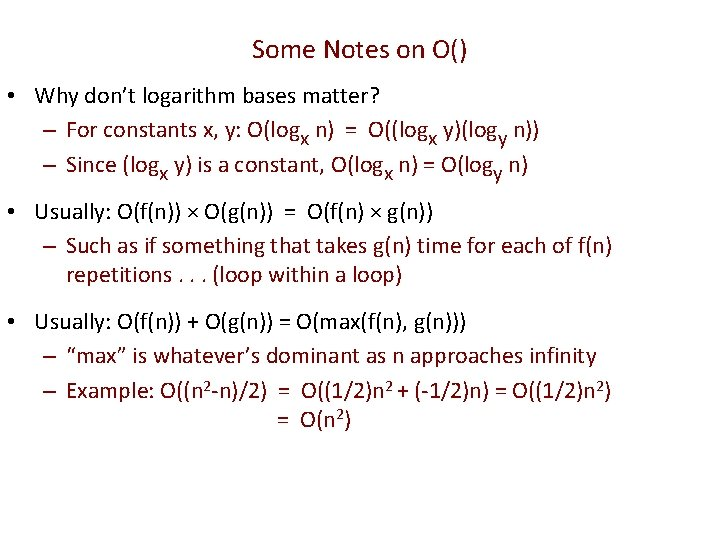Some Notes on O() • Why don’t logarithm bases matter? – For constants x, y: O(logx n) = O((logx y)(logy n)) – Since (logx y) is a constant, O(logx n) = O(logy n) • Usually: O(f(n)) × O(g(n)) = O(f(n) × g(n)) – Such as if something that takes g(n) time for each of f(n) repetitions. . . (loop within a loop) • Usually: O(f(n)) + O(g(n)) = O(max(f(n), g(n))) – “max” is whatever’s dominant as n approaches infinity – Example: O((n 2 -n)/2) = O((1/2)n 2 + (-1/2)n) = O((1/2)n 2) = O(n 2)runtimeof Merge. Sort /** Sort b[h. . k]. */ public static void m. S(Comparable[] b, int h, int k) { if (h >= k) return; Throughout, we int e= (h+k)/2; use m. S for m. S(b, h, e); merge. Sort, to m. S(b, e+1, k); make slides merge(b, h, e, k); easier to read } We will count the number of comparisons m. S makes Use T(n) for the number of array element comparisons that m. S makes on an array of size n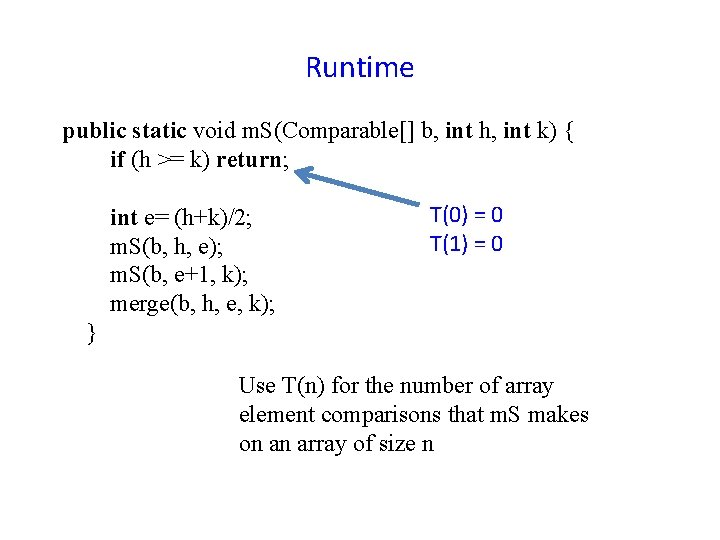Runtime public static void m. S(Comparable[] b, int h, int k) { if (h >= k) return; int e= (h+k)/2; m. S(b, h, e); m. S(b, e+1, k); merge(b, h, e, k); T(0) = 0 T(1) = 0 } Use T(n) for the number of array element comparisons that m. S makes on an array of size nRuntime public static void m. S(Comparable[] b, int h, int k) { if (h >= k) return; int e= (h+k)/2; m. S(b, h, e); m. S(b, e+1, k); merge(b, h, e, k); } Recursion: T(n) = 2 * T(n/2) + comparisons made in merge Simplify calculations: assume n is a power of 2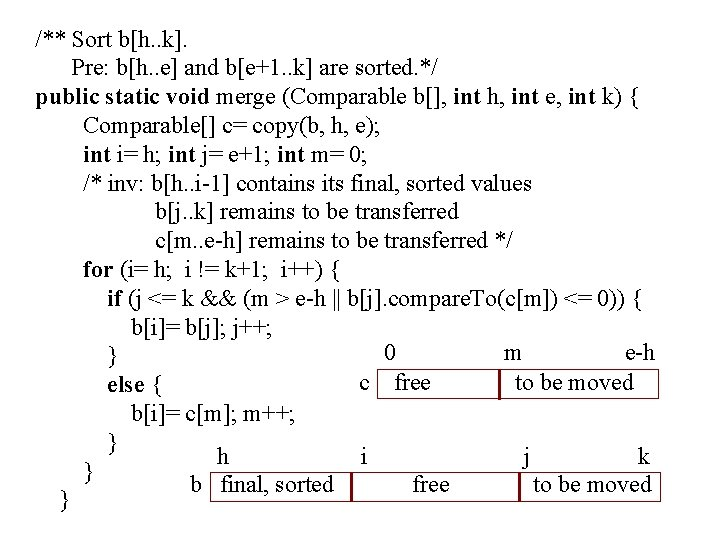/** Sort b[h. . k]. Pre: b[h. . e] and b[e+1. . k] are sorted. */ public static void merge (Comparable b[], int h, int e, int k) { Comparable[] c= copy(b, h, e); int i= h; int j= e+1; int m= 0; /* inv: b[h. . i-1] contains its final, sorted values b[j. . k] remains to be transferred c[m. . e-h] remains to be transferred */ for (i= h; i != k+1; i++) { if (j <= k && (m > e-h || b[j]. compare. To(c[m]) <= 0)) { b[i]= b[j]; j++; 0 m e-h } c free to be moved else { b[i]= c[m]; m++; } h i j k } b final, sorted free to be moved }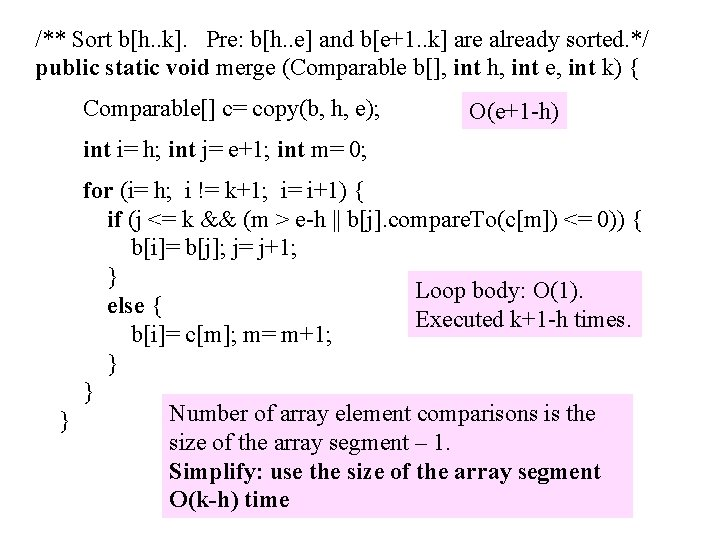/** Sort b[h. . k]. Pre: b[h. . e] and b[e+1. . k] are already sorted. */ public static void merge (Comparable b[], int h, int e, int k) { Comparable[] c= copy(b, h, e); O(e+1 -h) int i= h; int j= e+1; int m= 0; for (i= h; i != k+1; i= i+1) { if (j <= k && (m > e-h || b[j]. compare. To(c[m]) <= 0)) { b[i]= b[j]; j= j+1; } Loop body: O(1). else { Executed k+1 -h times. b[i]= c[m]; m= m+1; } } Number of array element comparisons is the } size of the array segment – 1. Simplify: use the size of the array segment O(k-h) timeRuntime We show to do an analysis, assuming n is a power of 2 (just to simplify the calculations) Use T(n) for number of array element comparisons to mergesort an array segment of size n public static void m. S(Comparable[] b, int h, int k) { if (h >= k) return; int e= (h+k)/2; m. S(b, h, e); T(e+1 -h) comparisons m. S(b, e+1, k); T(k-e) comparisons merge(b, h, e, k); (k+1 -h) comparisons } Thus: T(n) < 2 T(n/2) + n, with T(0) = 0, T(1) = 0Runtime Thus, for any n a power of 2, we have T(1) = 0 T(n) = 2*T(n/2) + n for n > 1 We can prove that T(n) = n lg n means log 2 n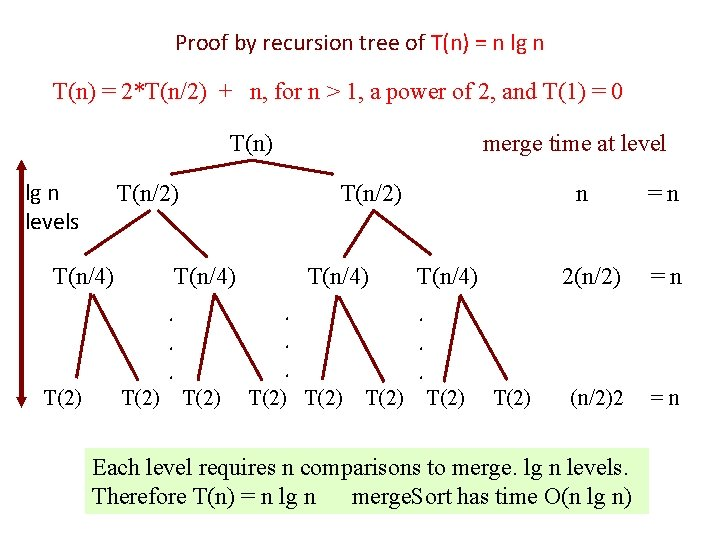Proof by recursion tree of T(n) = n lg n T(n) = 2*T(n/2) + n, for n > 1, a power of 2, and T(1) = 0 T(n) lg n levels T(n/2) T(n/4) T(2) T(n/4). . . T(2) merge time at level T(2) n T(n/4) =n 2(n/2) =n (n/2)2 =n . . . T(2) Each level requires n comparisons to merge. lg n levels. Therefore T(n) = n lg n merge. Sort has time O(n lg n)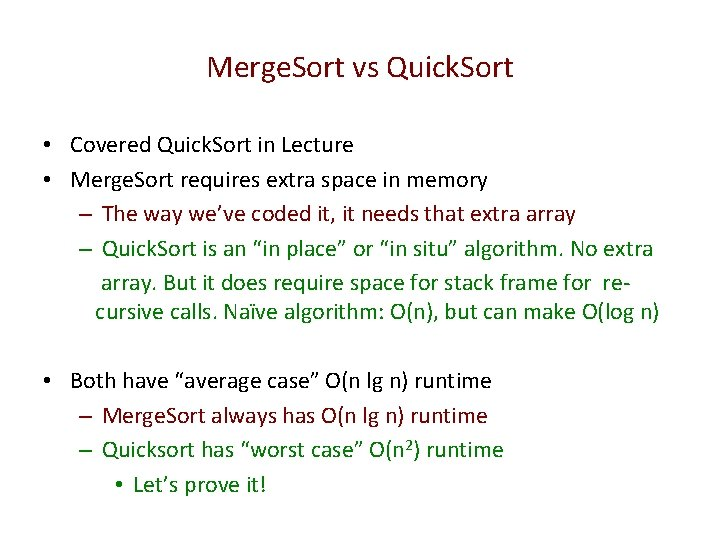Merge. Sort vs Quick. Sort • Covered Quick. Sort in Lecture • Merge. Sort requires extra space in memory – The way we’ve coded it, it needs that extra array – Quick. Sort is an “in place” or “in situ” algorithm. No extra array. But it does require space for stack frame for recursive calls. Naïve algorithm: O(n), but can make O(log n) • Both have “average case” O(n lg n) runtime – Merge. Sort always has O(n lg n) runtime – Quicksort has “worst case” O(n 2) runtime • Let’s prove it!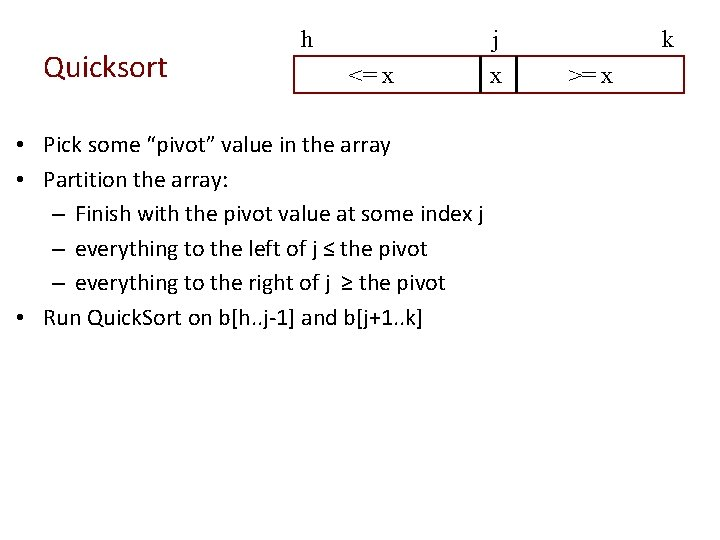Quicksort h j <= x • Pick some “pivot” value in the array • Partition the array: – Finish with the pivot value at some index j – everything to the left of j ≤ the pivot – everything to the right of j ≥ the pivot • Run Quick. Sort on b[h. . j-1] and b[j+1. . k] x k >= x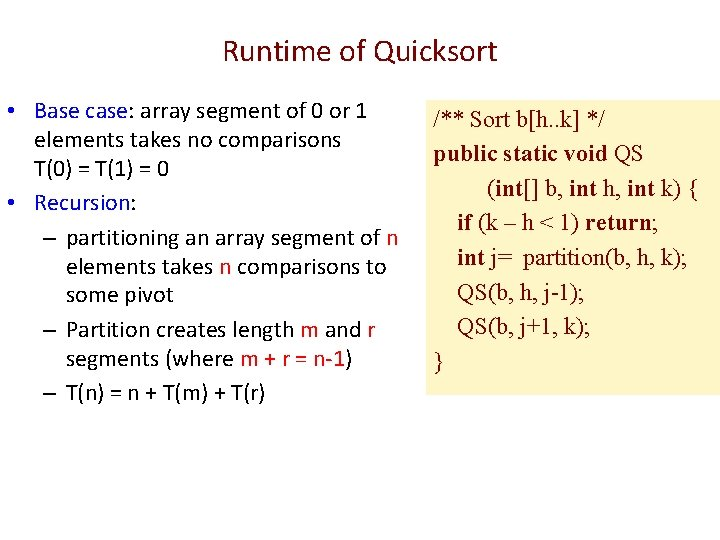Runtime of Quicksort • Base case: array segment of 0 or 1 elements takes no comparisons T(0) = T(1) = 0 • Recursion: – partitioning an array segment of n elements takes n comparisons to some pivot – Partition creates length m and r segments (where m + r = n-1) – T(n) = n + T(m) + T(r) /** Sort b[h. . k] */ public static void QS (int[] b, int h, int k) { if (k – h < 1) return; int j= partition(b, h, k); QS(b, h, j-1); QS(b, j+1, k); }Runtime of Quicksort • T(n) = n + T(m) + T(r) – Look familiar? • If m and r are balanced (m ≈ r ≈ (n-1)/2), we know T(n) = n lg n. • Other extreme: – m=n-1, r=0 – T(n) = n + T(n-1) + T(0) /** Sort b[h. . k] */ public static void QS (int[] b, int h, int k) { if (k – h < 1) return; int j= partition(b, h, k); QS(b, h, j-1); QS(b, j+1, k); }Worst Case Runtime of Quicksort When T(n) = n + T(n-1) + T(0) Hypothesis: T(n) = (n 2 – n)/2 Base Case: T(1) = (12 – 1)/2=0 Inductive Hypothesis: assume T(k)=(k 2 -k)/2 T(k+1) = k + (k 2 -k)/2 + 0 = (k 2+k)/2 = ((k+1)2 –(k+1))/2 • Therefore, for all n ≥ 1: T(n) = (n 2 – n)/2 = O(n 2) • • /** Sort b[h. . k] */ public static void QS (int[] b, int h, int k) { if (k – h < 1) return; int j= partition(b, h, k); QS(b, h, j-1); QS(b, j+1, k); }Worst Case Space of Quicksort You can see that in the worst case, the depth of recursion is O(n). Since each recursive call involves creating a new stack frame, which takes space, in the worst case, Quicksort takes space O(n). That is not good! /** Sort b[h. . k] */ public static void QS (int[] b, int h, int k) { if (k – h < 1) return; int j= partition(b, h, k); QS(b, h, j-1); QS(b, j+1, k); To get around this, rewrite Quick. Sort so that it is iterative but it sorts the smaller } of two segments recursively. It is easy to do. The implementation in the java class that is on the website shows this.